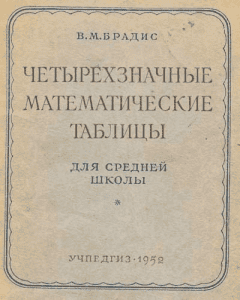## 3/11/2011

### Four-valued mathematical tables for blondes

Four-valued mathematical tables of Bradis - this was the basic mathematical reference book of soviet schoolchildren, students, engineers to appearance of calculators.

A trigonometric table for blondes is done by me to the navigation more informatively saturated in a plan. It what you did not lose way and did not entangle trigonometric functions.

Trigonometric table of sines and cosines - from 0 to 90 degrees punctually to the minute corner.

Trigonometric table tangent cotangent in degrees - from 0 to 90 degrees punctually to the minute corner.

Trigonometric table in radians - sin, cos, tan.

We will do justice to work of Bradis and we will remember a bit history. His book "Tables of the four-valued logarithms and natural trigonometric sizes" went out in 1921. This book was repeatedly reprinted, but already under more simple name "The Four-valued mathematical tables". This bestseller looked approximately so.Four-valued mathematical tables of Bradis

It is possible to say without a false modesty, that on these tables all Soviet Union was built, a man started to fly in space, a soviet nuclear club was created et cetera. Schoolchildren, engineers, scientists - all used the tables of Bradis. We will remember those distant times - the Internet is not present, mobile telephones are not present, computers and calculators are not present. Even televisions were not then! There were only books. In many books formulas were written for a calculation, and necessary for calculations numerical values were taken from the tables of Bradis. And what did numbers multiply by then? Not on accounts... Well and time was! As it was then possible normally to live??? But lived somehow.

An interesting question arises up. What mathematical tables did Americans build famous sky-scrapers on and created the nuclear club? In fact did not they steal for us tables of Bradis? Omniscient Wikipedia is quiet on this occasion, and information about the table of Bradis in Wikipedia in English language I did not find. There is there a mathematical reference book of Abramowitz, Milton and Irene A. Stegun with tables, but he is published was only in 1964. And that did Americans have to these tables?

I used the table of Bradis once. This whole art, to search the value of trigonometric functions punctually to the minute corner. Fortunately, today we have calculators.

Unfortunately, work of great soviet writer of mathematical tables Bradis me does not interest. Therefore the four-valued mathematical table into language of blondes transferred will not be. In the simplified kind the table of Bradis will be presented in the tables of sine, cosine, tangent and cotangent.

#### 1 comment:

1.Interesting to think that the Soviet Union was built on the mathematical tables of this one gentleman, and possibly the US too!